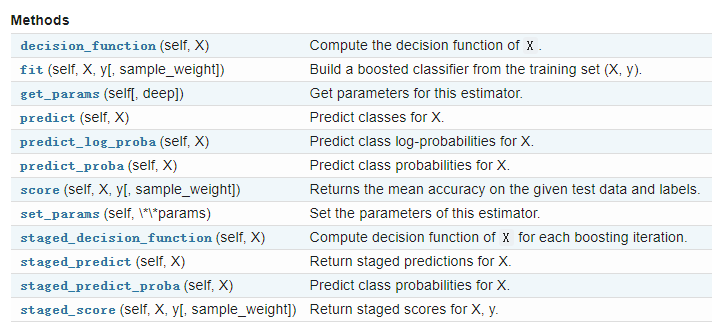class sklearn.ensemble.AdaBoostClassifier(base_estimator=None, n_estimators=50,
learning_rate=1.0, algorithm=’SAMME.R’,
random_state=None)


• n_estimators： 整数型，可选参数，默认为50。弱学习器的最大迭代次数，或者说最大的弱学习器的个数。一般来说n_estimators太小，容易欠拟合，n_estimators太大，又容易过拟合，一般选择一个适中的数值。默认是50。在实际调参的过程中，我们常常将n_estimators和下面介绍的参数learning_rate一起考虑。
• learning_rate： 浮点型，可选参数，默认为1.0。每个弱学习器的权重缩减系数，取值范围为0到1，对于同样的训练集拟合效果，较小的v意味着我们需要更多的弱学习器的迭代次数。通常我们用步长和迭代最大次数一起来决定算法的拟合效果。所以这两个参数n_estimators和learning_rate要一起调参。一般来说，可以从一个小一点的v开始调参，默认是1。
• random_state： 整数型，可选参数，默认为None。如果RandomState的实例，random_state是随机数生成器; 如果None，则随机数生成器是由np.random使用的RandomState实例。### 例子

>>> from sklearn.ensemble import AdaBoostClassifier
>>> from sklearn.datasets import make_classification
>>> X, y = make_classification(n_samples=1000, n_features=4,
...                            n_informative=2, n_redundant=0,
...                            random_state=0, shuffle=False)
>>> clf = AdaBoostClassifier(n_estimators=100, random_state=0)
>>> clf.fit(X, y)
learning_rate=1.0, n_estimators=100, random_state=0)
>>> clf.feature_importances_
array([0.28..., 0.42..., 0.14..., 0.16...])
>>> clf.predict([[0, 0, 0, 0]])
array()
>>> clf.score(X, y)
0.983...